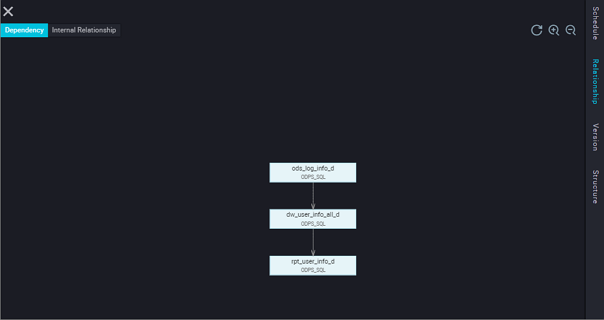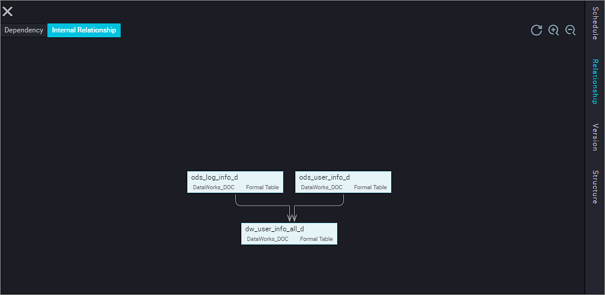This topic describes relationships that displays the relations between the current node and other nodes. This relationship displays two parts: The dependency diagram and the internal relationship diagram.

## Dependency graph

Depending on the node dependency, the dependency graph shows whether the current node dependency meets expectations. If the dependency graph does not meet expectations, you can return to the schedule configuration interface to reset.## Internal relationship diagram

The internal relationship diagram is parsed based on the node code, for example:

``````INSERT OVERWRITE TABLE dw_user_info_all_d PARTITION (dt='\${bdp.system.bizdate}')
SELECT COALESCE(a.uid, b.uid) AS uid
, b.gender
, b.age_range
, B. flavdiac
, a.region
, a.device
, a.identity
, a.method
, a.url
, a.referer
, a.time
FROM (
VALUES
From fig
WHERE dt = \${bdp.system.bizdate}
) a
LEFT OUTER JOIN (
VALUES
FROM ods_user_info_d
WHERE dt = \${bdp.system.bizdate}
) b
on a.uid = b.uid ;``````

According to the preceding SQL, the parsed internal relationship map join "dw_user_info_all_d" with "ods_log_info_d", and export table as follows :